# Volume of a Cube

The Volume of a Cube

A cube is a solid shape in which all 3-dimensional lengths are equal to each other. It, therefore, can be assumed that the length, breadth and heights are of the same dimension.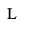The volume of a cube is length of a slide cubed.  Volume is measured in cubic units (in3,ft3,cm3,metc)

The volume V of a cube is given as…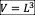Example 1:

Find the volume of the cube when the length of each side is 5cm.
Solution

Substitute L = 5 into the volume of a cube equation…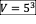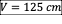Example 2:

Find the total volume of the cube shown…